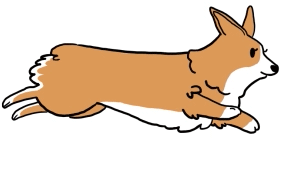IntroductionIntroduction# Multiplying and Dividing Negative Numbers

INTRO
When it comes to multiplying and dividing negative numbers, we like to fast forward and rewind to help us determine whether the final answer should be positive or negative.
Hear us out! 🙏🏽 Let’s say we rewind a cute video of a puppy running forward.### ⏮ RewindNotice the puppy now appears to be running backward. We can imagine that
• The original motion of the puppy matches the sign of the first number (forwards = positive, backwards = negative).
• The mode of the tv matches the sign of the second number (fast forward = positive, rewind = negative).
• The new motion of the puppy matches the sign of the final answer.
Play around with this TV to test out which combos give us positive numbers and which give us negative numbers.Check out our
Calculator
or explore our
Lesson
and
Practice

CALCULATOR

KEY STEPS

## How to Multiply and Divide Negative Numbers

First number is and second number is.

### Step 1. Imagine the first number.

If the first number is positive, imagine a video of someone running forward.

### Step 2. Imagine the second number.

If the second number is positive, imagine rewinding the video of someone running from Step 1.

### Step 3. Determine the sign of the final answer.

A video of someone running forward that we rewind will look like a video of someone running backward. So our answer is negative.### ⏮ Rewind### Step 4. Multiply/divide the numbers.

Determine the product/quotient of the numbers. Add the sign if the answer should be negative based on Step 3.
LESSON
Multiplying Negative Numbers

## Multiplying Negative Numbers

When we multiply negative numbers, we use the fast forward and rewind hack to determine the sign of the final answer before calculating the product.
 Problem Imagine positive × negative = negative forward motion and rewind = backward motion negative × positive = negative backward motion and fast forward = backward motion negative × negative = positive backward motion and rewind = forward motion
Let's walk through a couple examples to see this in action!
PRACTICE
Multiplying Negative Numbers

## Practice: Multiplying Negative Numbers

Question 1 of 4:

### Step 1. Imagine the first number.

Is the first number positive or negative?

LESSON
Dividing Negative Numbers

## Dividing Negative Numbers

Our rules for dividing are the same as our rules for multiplying:
 Problem Imagine positive ÷ negative = negative forward motion and rewind = backward motion negative ÷ positive = negative backward motion and fast forward = backward motion negative ÷ negative = positive backward motion and rewind = forward motion
Let's walk through a couple examples to see this in action!
PRACTICE
Dividing Negative Numbers

## Practice: Dividing Negative Numbers

Question 1 of 4:

### Step 1. Imagine the first number.

Is the first number positive or negative?

CONCLUSION
Dang, look at you go! Thanks for checking out this lesson ☺️🙏. Where to next?Leave Feedback